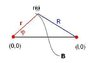# Highly Challenging Optics Problem

• bobquantum

## Homework Statement

A point source of light P that is located in a medium 1 with refractive index n1, and its
image O in the medium 2 with refractive index n2 > n1. The
boundary between the two media crosses the plane of the drawing along the curve B. Taking the position of the image as an origin for the Cartesian system of coordinates (x, y), the position of the source can be defined as l on the axis x, and the position of the crosspoint C between the surface B and the axis x as f0. In the 2D – problem, the position of P becomes (l, 0), and the position of C becomes (f0, 0). Basing on the Fermat’s principle, find the equation of the boundary curve B as a function y(x), for particular l and f0.

## Homework Equations

Fermat's Principle: Light always travels the path of least time.
Optical length: n1 * l1 = n2 * l2 given two mediums with refractive indexes of n1 and n2, an the length of the light path l1 and l2, respectively.

## The Attempt at a Solution

I have attempted to set up the equation as follows.

Now, we can use f0 and l to set up the optical length. By Fermat's Principle, we should have the optical length of every other path equal to this optical length, which is (l-f0) * n1 + f0 * n2

Let a point X(x, y) be on the boundary curve B. Thus, using the distance formula, we can set up the following equation:

n1 * sqrt ((l-x) ^2 + y^2) + n2 * sqrt (x^2 + y^2) = (l-f0) *n1 + f0 * n2.

The problem is, I cannot solve this equation. I have tried Wolfram Alpha, but it cannot solve for this equation in terms of x. PLEASE HELP ALL PHYSICS GENIUSES.

Thank you,
Bobquantum

ehild

Last edited:
Show the figure please. How does the curve B look like? You need the equation of the curve for the solution.

ehild

Isn't the equation of the curve the thing you are meant to solve for?

Oh sorry, I have not read the whole problem, just missed a figure to understand the problem.

Those two square roots are scary. Try to do it in polar coordinates. You get R with the Cosine Law, and then solve the equation n1R+n2r=n1(L-f0)+n2f0 for r in terms of φ.

ehild

#### Attachments

•fermat.JPG
5 KB · Views: 421
Last edited:
The problem is, I am not allowed to introduce more variables (i.e. no radius of curvature or angles). I must solve using the variables given. I wonder if there is a simpler way to represent x and y in a function. This is a POPTOR question by the way, but the deadline is past, so you guys don't have to worry about violating any rules. Just wanted to know if I did it correctly.

Your equation is correct, it is only terrible to solve it that way. It would mean moving one square root to the other side, then squaring the whole equation, then isolating the remaining square root, and squaring the equation again.
When using polar coordinates, you represent x by x=r cos(phi) and y by y=r sin(phi). They are just notations, to make the solution easier. If you get the function r(phi) you can transform back to x and y. But it is still a very complicated thing.
I could not find any simpler method. Anyway, your equation is an implicit definition of y(x) if you replace y by y(x).I outline the solution in terms of r and phi.

$$n_2r +n_1R=n_1(L-f_0)+n_2f_0$$
$$R^2=r^2+L^2-2rRcos(\phi)$$
Isolate R from the first eq.

$$R=L+(n_2/n_1-1)f_0- n_2/n_1r$$

Introduce the notations n2/n1=n (it is the refractive index of medium 2 with respect to medium 1) and $L+(n_2/n_1-1)f_0=A$, and substitute for R into the second equation
$$(A-nr)^2=L^2+r^2-2rLcos(\phi)$$.

$$r^2(n^2-1)-2r(An-Lcos(\phi)+(A^2-L^2)=0$$

I calculated the curve for n=1.4, L=1, fo=0.5.

ehild

Last edited: